# Propose a plausible two-step mechanism for the given reaction (Pool: 5 of 5) Propose a plausible...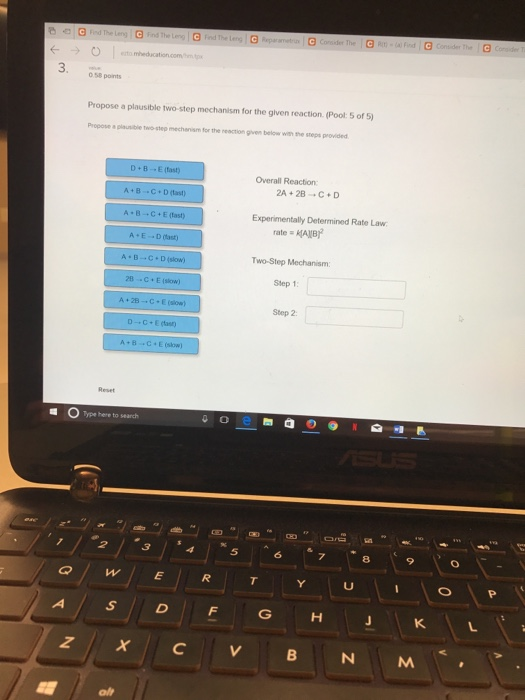Propose a plausible two-step mechanism for the given reaction (Pool: 5 of 5) Propose a plausible two-step mechanism for the reaction given below with the steps provided. Overall Reaction: 2A + 2B rightarrow C + D Experimentally Determined Rate Law: rate = K[A][B]^2 Two-Step Mechanism: Step 1: Step 2: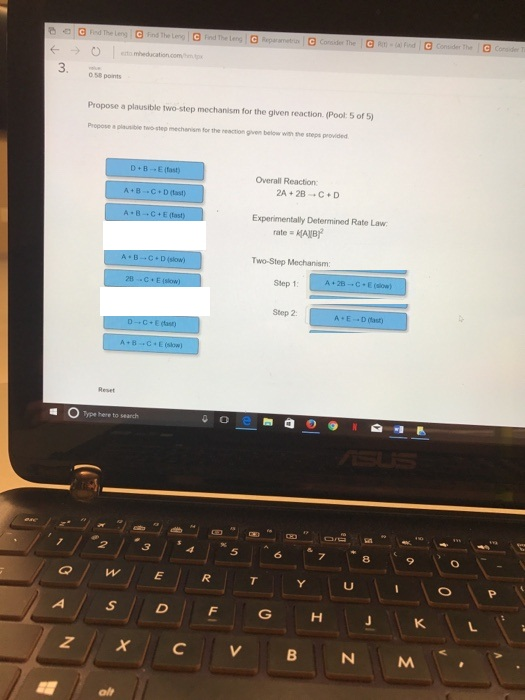##### Add Answer to: Propose a plausible two-step mechanism for the given reaction (Pool: 5 of 5) Propose a plausible...
Similar Homework Help Questions
• ### select all of the mechanisms that are plausible for a given reaction and it's experimentally determined...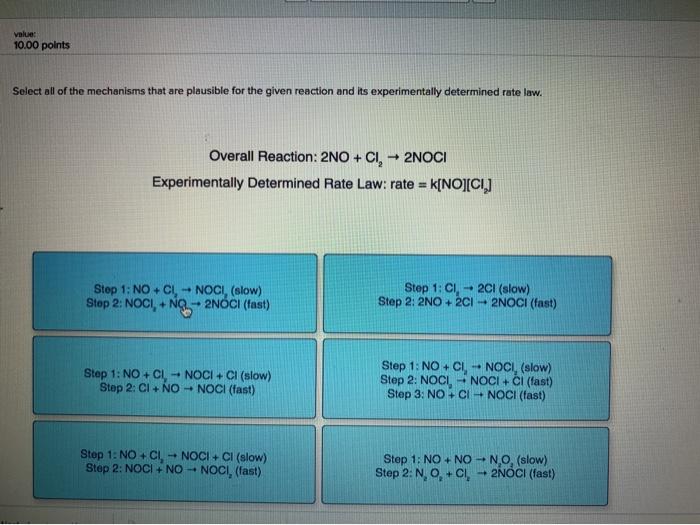select all of the mechanisms that are plausible for a given reaction and it's experimentally determined the rate law. 10.00 points Select all of the mechanisms that are plausible for the given reaction and its experimentally determined rate law. Overall Reaction: 2NO + CI, + 2NOCI Experimentally Determined Rate Law: rate = k[NO][CI] Step 1: NOCI, + NOCI, (slow) Step 2: NOCI, NQ -- 2NÓCI (fast) Step 1: CI, -+ 2Cl (slow) Step 2: 2NO+2CI2NOCI (fast) Step 1: NOCI, NOCI...

• ### Given the following proposed mechanism, predict the rate law for the overall reaction: . A2 A...

Given the following proposed mechanism, predict the rate law for the overall reaction: . A2 A 2 + 2B B → → 2AB A B (overall reaction) Mechanism A2⇌ A 2 ⇌ 2A A fast A A + B B → → AB A B slow Given the following proposed mechanism, predict the rate law for the overall reaction: . + 2 2 (overall reaction) Mechanism 2 fast + slow Rate = k[A2]2[B]1/2 k [ A 2 ] 2 [...

• ### What two criteria must a plausible mechanism meet? Select both criteria in the options. elementary steps...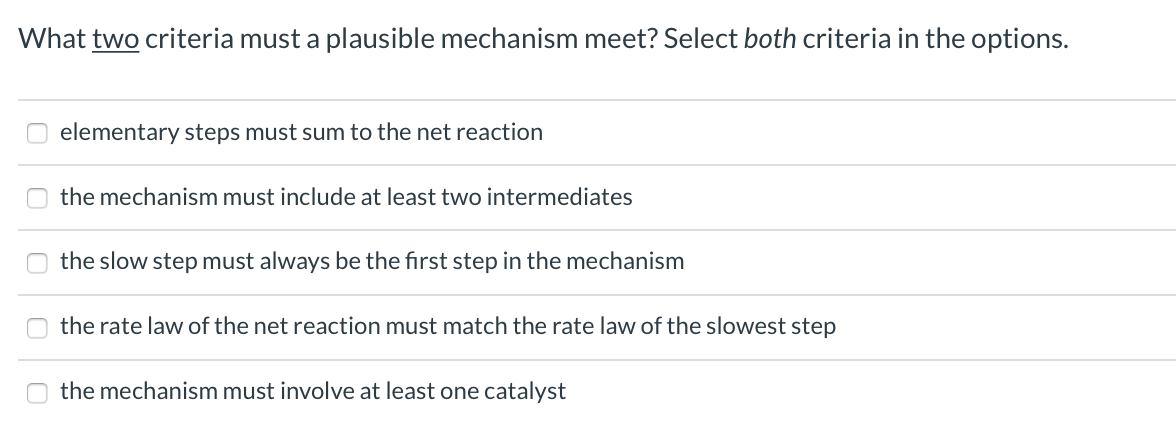What two criteria must a plausible mechanism meet? Select both criteria in the options. elementary steps must sum to the net reaction the mechanism must include at least two intermediates the slow step must always be the first step in the mechanism the rate law of the net reaction must match the rate law of the slowest step the mechanism must involve at least one catalyst Areaction profile is shown. Which step is the rate limiting (or rate determining) step...

• ### Consider the mechanism. Step 1: Step 2: Overall: 2A B B+C HD 2A+ C D equilibrium...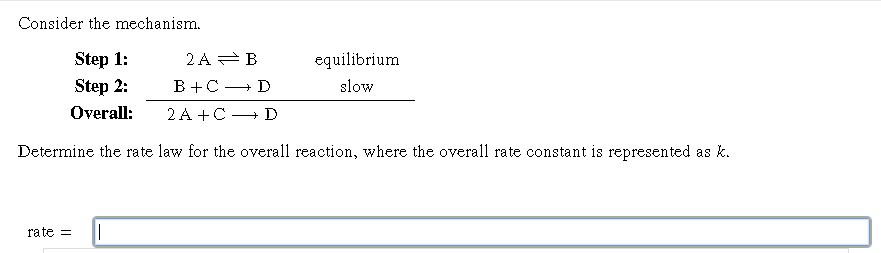Consider the mechanism. Step 1: Step 2: Overall: 2A B B+C HD 2A+ C D equilibrium slow Determine the rate law for the overall reaction, where the overall rate constant is represented as k. rate = 1

• ### Consider the mechanism. 2A - B slow Step 1: Step 2: Overall: B + C -→...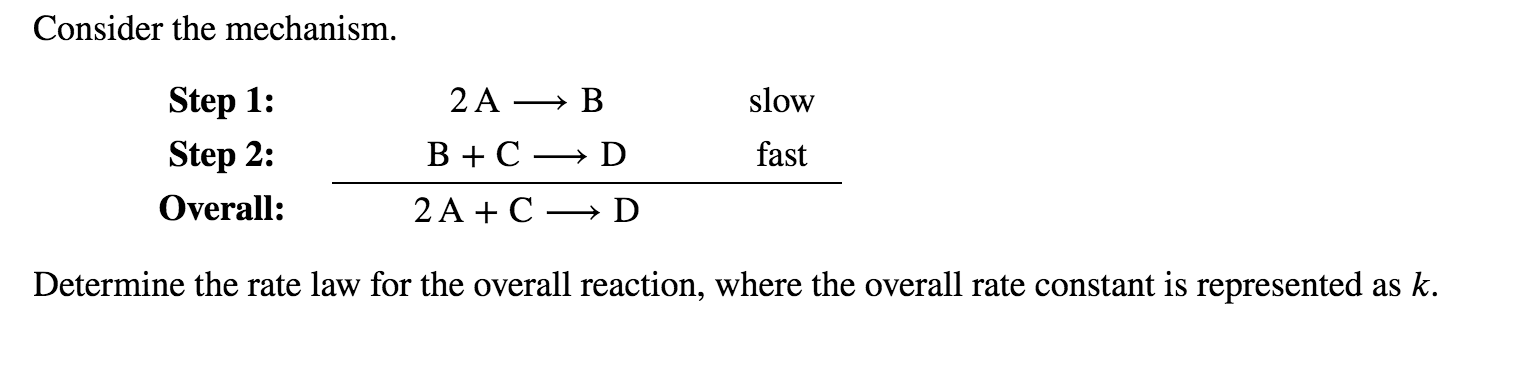Consider the mechanism. 2A - B slow Step 1: Step 2: Overall: B + C -→ D fast 2 A + C -- D Determine the rate law for the overall reaction, where the overall rate constant is represented as k.

• ### A proposed mechanism for the reaction of NO_2 and CO is 2 NO_2(g) rightarrow NO(g) +...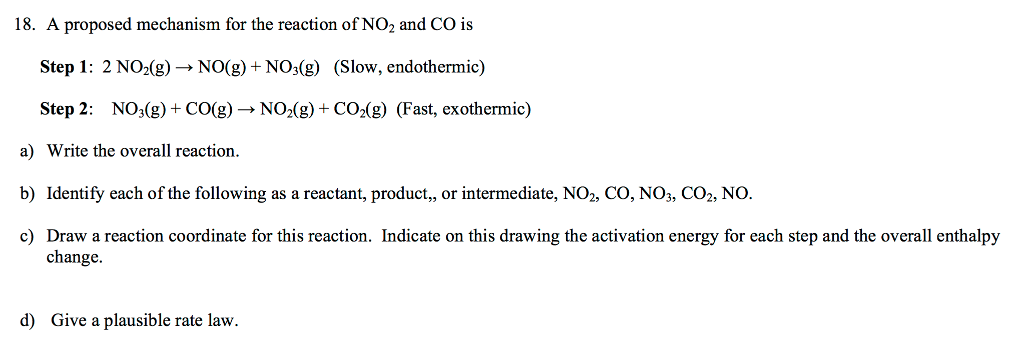A proposed mechanism for the reaction of NO_2 and CO is 2 NO_2(g) rightarrow NO(g) + NO_3(g) (Slow, endothermic) NO_3(g) + CO(g) rightarrow NO_2(g) + CO_2(g) (Fast, exothermic) a) Write the overall reaction. b) Identify each of the following as a reactant, product, , or intermediate, NO_2, CO, NO_3, CO_2, NO. c) Draw a reaction coordinate for this reaction. Indicate on this drawing the activation energy for each step and the overall enthalpy change. d) Give a plausible rate law.

• ### Consider the following mechanism. step 1 2A > B slow B+ C D fast step 2 overall: 2A+C D Determine the rate law for t...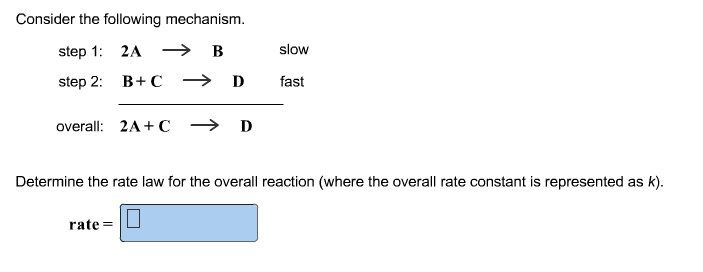Consider the following mechanism. step 1 2A > B slow B+ C D fast step 2 overall: 2A+C D Determine the rate law for the overall reaction (where the overall rate constant is represented as k) rate=

• ### Consider the following mechanism. step 1: A+B C step 2: C+A → D equilibrium slow overall: 2A+B - D Determine the rate l...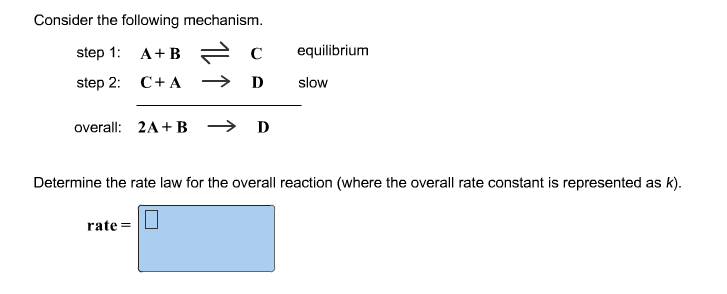Consider the following mechanism. step 1: A+B C step 2: C+A → D equilibrium slow overall: 2A+B - D Determine the rate law for the overall reaction (where the overall rate constant is represented as k). rate=

• ### Seat # Ver. K J. Quest. 6 (9 pts). The following initial rates were obtained for the reaction -120 kJ Δ Η 2C 2A +B [B] (M) 0.30 0.60 [A] (M) 0.20 Rate (M/s) 0.127 0.40 1.02 0.254 0.509 0.60 0.20 0...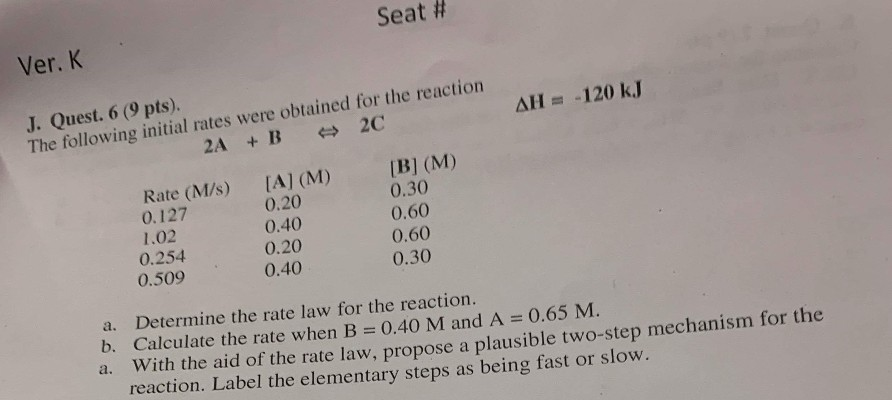Seat # Ver. K J. Quest. 6 (9 pts). The following initial rates were obtained for the reaction -120 kJ Δ Η 2C 2A +B [B] (M) 0.30 0.60 [A] (M) 0.20 Rate (M/s) 0.127 0.40 1.02 0.254 0.509 0.60 0.20 0.40 0.30 a. Determine the rate law for the reaction. b. Calculate the rate when B 0.40 M and A 0.65 M. a. With the aid of the rate law, propose a plausible two-step mechanism for the reaction. Label...

• ### Consider the overall reaction and proposed mechanism. step 1: A + BC --> AB + C...

Consider the overall reaction and proposed mechanism. step 1: A + BC --> AB + C step 2: AB + D --> AD + B step 3: B + C --> BC overall reaction: A + D --> AD What step is rate determining (slow) if the rate law of overall reaction is Rate = k[A][BC]

Free Homework App